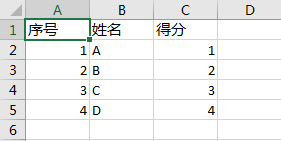﻿ 如何用vba将excel单元格区域转化为一维数组？ - EXCEL VBA - ExcelOffice【微信公众号：水星Excel】

# 如何用vba将excel单元格区域转化为一维数组？```Sub QQ1722187970()
Dim arr()
Dim oWK As Worksheet
Set oWK = Sheet1
Dim oRng As Range
Set oRng = oWK.Range("a1:c5")
arr = oRng.Value
End Sub``````Sub QQ1722187970()
Dim arr()
Dim arrTemp()
Dim oWK As Worksheet
Set oWK = Sheet1
Dim oRng As Range
'单元格区域的第一列
Set oRng = oWK.Range("a1:a5")
For i = 1 To 3
arr = oRng.Offset(0, i - 1).Value
'1次转置即可转化为一维数组
arrTemp = Excel.Application.WorksheetFunction.Transpose(arr)
Next i
End Sub```

```Sub QQ1722187970()
Dim arr()
Dim arrTemp()
Dim oWK As Worksheet
Set oWK = Sheet1
Dim oRng As Range
'单元格区域的第一行
Set oRng = oWK.Range("a1:c1")
For i = 1 To 3
arr = oRng.Offset(0, i - 1).Value
'2次转置才能转化为一维数组
arrTemp = Excel.Application.WorksheetFunction.Transpose(Excel.Application.WorksheetFunction.Transpose(arr))
Next i
End Sub
```